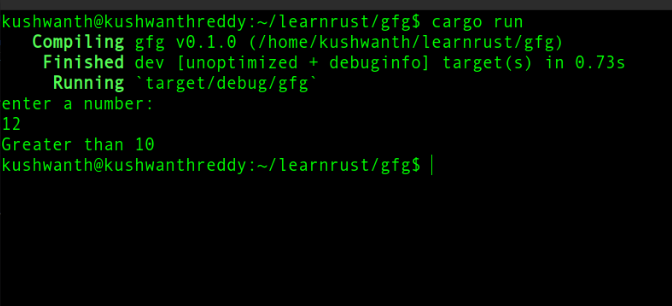Open In App

# Conditionals in Rust

Conditional Statements are the decision-making statements based on given conditions. Conditional statements are common in programming languages and rust has them and unlike many of them, the boolean condition doesn’t need to be surrounded by parentheses but is better to use, and each condition is followed by a block. Some things to keep in mind while using conditional statements.

• Always compare similar data types.
• The conditional statement always returns a boolean value.

Conditional statements supported by Rust are

• if statement
• else if statement
• else statement

Syntax:

```fn main() {
if condition1 {
// executed if condition1 true
statement;
}
else if condition2 {
// executed if condition2 is true
statement;
}
else{
// executed if both condition1 and condition2 are false
statement;
}
}```

### If statement

If statement is the first basic condition that needs to be checked, In other words, you provide a condition and then state, “If this condition is met, run this block of code. If the condition is not met, do not run this block of code.”

Example:

A sample code is below which checks whether the given number is greater than 10 or not. Here the below approach is used:

• Provide an integer input.
• The given input is checked whether is greater than 10
• If true “greater than 10” is printed as output
• If false the program is exited.

## Rust

 `use` `std::io;`` ` `fn` `main() {``    ``println!(``"enter a number:"``);``    ``let` `mut` `strn = String::new();``    ``io::stdin()``        ``.read_line(&``mut` `strn)``        ``.expect(``"failed to read input."``);`` ` `    ``let` `n: i32 =  strn.trim().parse().expect(``"invalid input"``);`` ` `    ``if` `n>10 {``        ``println!(``"Greater than 10"``); }``}`

Output:### Else statement

An if statement will be executed when the given condition is true, Else statement is executed when the given condition fails.

Example 1: A simple program to check the string input is equal to “gfg”.

## Rust

 `fn` `main() {`` ` `    ``let` `strn = ``"Geeksforgeeks"``;``    ``let` `fixed_string = ``"gfg"``;`` ` ` ` `    ``if` `fixed_string==strn {``        ``println!(``"Same"``); ``    ``}``else``{``        ``println!(``"Not Same"``);``    ``}``}`

Output:

`Not Same`

Example 2: Program to check whether the given number is greater than 10 or not.

## Rust

 `fn` `main() {`` ` `    ``let` `n = 7;`` ` `    ``if` `n>10 {``        ``println!(``"Greater than 10"``); ``    ``}``else``{``        ``println!(``"Smaller than 10"``);``    ``}``}`

Output:

`Smaller than 10`

### Else if statement

Else if statement is used to check another condition after the if condition before the else condition. A program can have as many else if statements but can only have one if and one else statements.

Example: The below program checks whether the value is smaller or equals to or greater than 10.

## Rust

 `fn` `main() {`` ` `    ``let` `n = 10;`` ` `    ``if` `n>10 {``        ``println!(``"Greater than 10"``);``    ``}``else` `if` `n==10 {``        ``println!(``"Equal to 10"``);``    ``}``else` `{``        ``println!(``"Smaller than 10"``);``    ``}``}`

Output:

`Equal to 10`